# Equation + arithmetic progression - math problems

#### Number of problems found: 61

• InsertInsert five numbers between 8 and 27 such numbers that, with two given ones, they form the first seven members of the geometric sequence.
• In the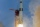In the arithmetic sequence a1 = 4.8, d = 0.4. How many consecutive members, starting with the first, need to be added so that the sum is greater than 170?
• The perimeterThe perimeter of the triangle is 24, the sides are integers and form an arithmetic sequence. Specify the side sizes of this triangle.
• Harry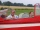Harry Thomson bought a large land in the shape of a rectangle with a circumference of 90 meters. He divided it into three rectangular plots. The shorter side has all three plots of equal length, their longer sides are three consecutive natural numbers. Fi
• Arithmetic progression 2The 3rd term of an Arithmetic progression is ten more than the first term, while the fifth term is 15 more than the second term. Find the sum of the 8th and 15th terms of the Arithmetic progression if the 7th term is seven times the first term.
• The sidesThe sides of a right triangle form an arithmetic sequence. The hypotenuse is 24 cm long. Determine the remaining sides of the triangle.
• Sequences AP + GPThe three numbers that make up the arithmetic sequence have the sum of 30. If we subtract from the first 5, from the second 4 and keep the third, we get the geometric sequence. Find AP and GP members.
• Squirrels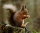The squirrels discovered a bush with hazelnuts. The first squirrel plucked one nut, the second squirrel two nuts, the third squirrel three nuts. Each new squirrel always tore one nut more than the previous squirrel. When they plucked all the nuts from the
• Annual growthThe population has grown from 25,000 to 33,600 in 10 years. Calculate what was the average annual population growth in%?
• Angles of a hexagon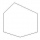Find the interior angles of a hexagon if the sizes of the angles form an arithmetic sequence, and the smallest angle is 70°.
• Find d 2Find d in an A. P. whose 5th term is 18 and 39th term is 120.
• 1 pageOne page is torn from the book. The sum of the page numbers of all the remaining pages is 15,000. What numbers did the pages have on the page that was torn from the book?
• Finite arithmetic sequenceHow many numbers should be inserted between the numbers 1 and 25 so that all numbers create a finite arithmetic sequence and that the sum of all members of this group is 117?
• SavingsThe depositor regularly wants to invest the same amount of money in the financial institution at the beginning of the year and wants to save 10,000 euros at the end of the tenth year. What amount should he deposit if the annual interest rate for the annua
• Volume of woodEvery year, at the same time, an increase in the volume of wood in the forest is measured. The increase is regularly p% compared to the previous year. If in 10 years the volume of wood has increased by 10%, what is the number p?
• Growth of woodThe annual growth of wood in the forest is estimated at 2%. In how many years will make the forest volume double?
• Pilsen circus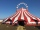The arrival of the circus in Pilsen was seen in the morning at 08:00 by a city citizen. He passed this information on 08:15 to three other residents of the city. Each of these three people then informed the other three residents at 08:30, and again at 08:
• AM of three numbersThe number 2010 can be written as the sum of 3 consecutive natural numbers. Determine the arithmetic mean of these numbers.
• Geometric progressiobIf the sum of four consective terms of geometric progression is 80 and arithmetic mean of second and fourth term is 30 then find terms?
• Pine's forest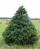There were so many pines in the forest that they were sequentially numbered 1, 2, 3,..., would use three times more digits than the pine trees alone. How many pine trees were there in the forest?

Do you have an exciting math question or word problem that you can't solve? Ask a question or post a math problem, and we can try to solve it.

We will send a solution to your e-mail address. Solved examples are also published here. Please enter the e-mail correctly and check whether you don't have a full mailbox.

Do you have a linear equation or system of equations and looking for its solution? Or do you have a quadratic equation? Equations Math problems. Arithmetic progression - math problems.